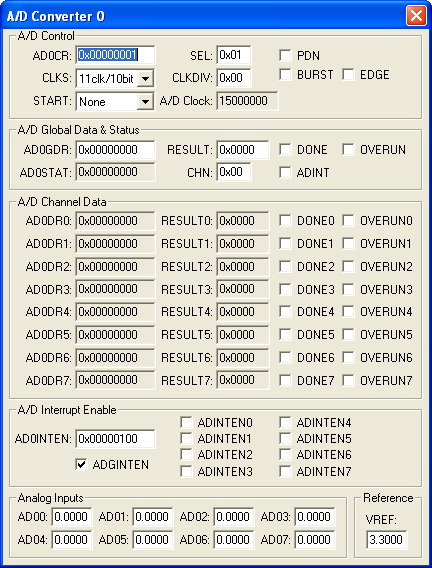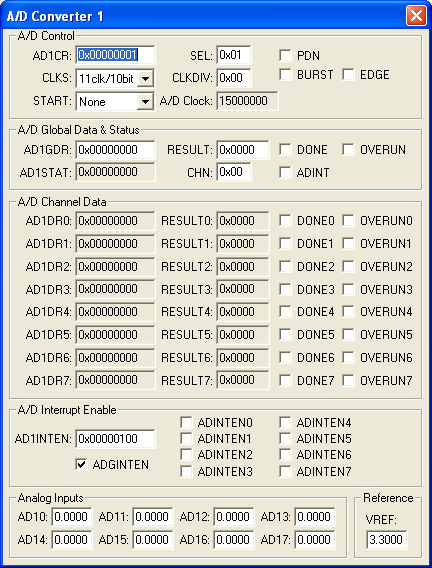Products Download Events Support Videos All Product Families ARM7, ARM9, and Cortex-M3 Products C16x, XC16x, and ST10 Products C251 and 80C251 Products Cx51 and 8051 Products Modified Anytime In the Last 7 Days In the Last 14 Days In the Last 30 Days In the Last 90 Days In the Last 6 Months

# Peripheral Simulation

For NXP (founded by Philips) LPC2138/01 — A/D Converters 0-1 (8-Channels)

Simulation support for this peripheral or feature is comprised of:

• Dialog boxes which display and allow you to change peripheral configuration.
• VTREGs (Virtual Target Registers) which support I/O with the peripheral.

These simulation capabilities are described below.

### A/D Converter 0 DialogThe A/D Converter dialog displays the status registers for all of the A/D converters supported by this device. The Analog Input Channels, listed at the bottom of the dialog, allow you to specify the voltage for each analog input.

A/D Control

• ADnCR (A/D n Control Register) contains the following control bits for the A/D controller:
• CLKS (Conversion Clocks)selects the number of clocks used for each conversion in Burst mode and the bit accuracy.
• START controls when and if the an A/D conversion is started.
• SEL selects which pins of the analog input (AINn) are to be sampled and converted.
• CLKDIV (Clock Divisor) is the value (minus 1) divided into the VPB clock to produce the A/D Rate.
• A/D Clock displays the A/D clock rate.
• PDN (Power Down) is set if the A/D is operational. When reset, the A/D is in power down mode.
• BURST is set to use the number of clocks specified in BURST. If reset, conversions are software controlled and requires 11 clocks.
• EDGE is set to start the conversion on a rising edge. If reset, conversion starts on a falling edge.

A/D Global Data & Status

• ADnGDR (A/D n Global Data Register) contains the following bits for controlling A/D data conversion:
• RESULT (Result Value) when DONE is set, contains the voltage input divided by the voltage reference.
• CHN (Channel) is the channel containing the converted bits.
• DONE is set when the A/D conversion completes.
• ADINT is set when an A/D interrupt occurs.
• OVERRUN is set if one or more conversions were overwritten before the current conversion completed.

A/D Channel Data

• ADnDR 0-7 (A/D n Channel Data Registers 0-7) contain the RESULT, DONE and OVERRUN status for each of the eight channels.

A/D Interrupt Enable

• ADnINTEN 0-7 (A/D n Channel Interrupt Enable 0-7) contains the interrupt enable bit for each of the eight channels(ADINTEN0 - ADINTEN7).
• ADGINTEN (A/D Global Interrupt Enable) is set to allow only the DONE flag to trigger and ADC complete interrupt. When reset, each channel enabled in ADnINTEN can trigger and ADC complete interrupt.

• ADn (Analog Data Input Channels n) are used for setting voltage input to the ADC. These inputs are alternate functions for Port pins. The text box displays the analog voltage value for each input channel.

### A/D Converter 1 DialogThe A/D Converter dialog displays the status registers for all of the A/D converters supported by this device. The Analog Input Channels, listed at the bottom of the dialog, allow you to specify the voltage for each analog input.

A/D Control

• ADnCR (A/D n Control Register) contains the following control bits for the A/D controller:
• CLKS (Conversion Clocks)selects the number of clocks used for each conversion in Burst mode and the bit accuracy.
• START controls when and if the an A/D conversion is started.
• SEL selects which pins of the analog input (AINn) are to be sampled and converted.
• CLKDIV (Clock Divisor) is the value (minus 1) divided into the VPB clock to produce the A/D Rate.
• A/D Clock displays the A/D clock rate.
• PDN (Power Down) is set if the A/D is operational. When reset, the A/D is in power down mode.
• BURST is set to use the number of clocks specified in BURST. If reset, conversions are software controlled and requires 11 clocks.
• EDGE is set to start the conversion on a rising edge. If reset, conversion starts on a falling edge.

A/D Global Data & Status

• ADnGDR (A/D n Global Data Register) contains the following bits for controlling A/D data conversion:
• RESULT (Result Value) when DONE is set, contains the voltage input divided by the voltage reference.
• CHN (Channel) is the channel containing the converted bits.
• DONE is set when the A/D conversion completes.
• ADINT is set when an A/D interrupt occurs.
• OVERRUN is set if one or more conversions were overwritten before the current conversion completed.

A/D Channel Data

• ADnDR 0-7 (A/D n Channel Data Registers 0-7) contain the RESULT, DONE and OVERRUN status for each of the eight channels.

A/D Interrupt Enable

• ADnINTEN 0-7 (A/D n Channel Interrupt Enable 0-7) contains the interrupt enable bit for each of the eight channels(ADINTEN0 - ADINTEN7).
• ADGINTEN (A/D Global Interrupt Enable) is set to allow only the DONE flag to trigger and ADC complete interrupt. When reset, each channel enabled in ADnINTEN can trigger and ADC complete interrupt.

• ADn (Analog Data Input Channels n) are used for setting voltage input to the ADC. These inputs are alternate functions for Port pins. The text box displays the analog voltage value for each input channel.

### A/D Global Start DialogThe A/D Global Start dialog is used to display and control simultaneous conversion activity on both A/D controllers.

A/D Control

• ADGSR (A/D Global Start Register) contains the following control bits for the A/D controller:
• START (START Mode) controls if and when an A/D conversion is started.
• BURST (BURST Mode) is set to allow continuous A/D conversions. the START mode must be 0.
• EDGE (EDGE Mode) is set to start conversion on a falling edge of the CAPn or MATn signal. If reset, conversion starts on a rising edge of these signals.

```AD0 = 0.000  /* Analog Input 0 = 0.000 volts */
```

You may create a debugger signal function to periodically change the value of the A/D input. The following signal function increases the A/D Channel 1 input voltage by 0.1 volts each second.

```signal void AD1_sig (void) {
float f;

for (f = 0.0; f < 5.0; f += 0.1)
{
swatch (1.0);                  // Delay 1 second
}
}
```